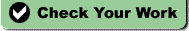# Introduction Quiz

 Name: Introduction Quiz

True/False
Indicate whether the sentence or statement is true or false.1.
Refer to the illustration above. Reproduction ensures the ongoing success of both species.
2.
Refer to the illustration above. The sand dollar and paramecium both show organization.
3.
Scientists have not discovered any new species on Earth in more than 20 years.
4.
Publication of the results of scientific investigations enables other scientists to verify these results.
5.
Resolution is a microscope’s power to increase an object’s apparent size.

Multiple Choice
Identify the letter of the choice that best completes the statement or answers the question.
6.
Biology is the study of
 a. minerals. c. the weather. b. life. d. energy.
7.
Homeostasis means
 a. a change over long periods of time. c. rapid change. b. keeping things the same. d. the same thing as evolution.
8.
Which of the following is a means by which heterotrophs can obtain energy?
 a. using water, carbon dioxide, and energy from the sun to produce sugars b. using water and carbon dioxide to produce energy-rich compounds c. consuming autotrophs d. consuming simple chemicals from the environment and using them to assemble complex chemicals and structures needed by the organism
9.
Which of the following is not necessarily a distinct property of living things?
 a. homeostasis c. complexity b. metabolism d. reproduction
10.
All organisms are composed of
 a. diatoms. c. cells. b. cellulose. d. None of the above
11.
Which example of scientific methodology is incorrect?
 a. Observation—A number of people in Zaire dying of a disease outbreak b. Measurement—A record of the number of people with symptoms of the disease and the number of people who had died from the disease c. Analysis of data—Comparison of the effects of mixing monkey cells with virus-containing blood in test tubes and the effects of mixing of liquid from these test tubes with fresh monkey cells d. Inference making—Identification of the Ebola virus as the cause of the disease by taking electron micrographs of substances found in the blood of persons affected with the disease
12.
Scientific hypotheses are most often tested by the process of
 a. communicating. c. experimenting. b. inferring. d. analyzing data.
13.
A hypothesis is
 a. a definite answer to a given problem. b. a testable possible explanation of an observation. c. a proven statement. d. a concluding statement.
14.
A hypothesis that does not explain an observation
 a. is known as an inaccurate forecast. c. is rejected. b. often predicts a different observation. d. None of the above
15.
A scientific theory
 a. is absolutely certain. b. is unchangeable. c. may be revised as new evidence is presented. d. is a controlled experiment.
16.
observation : hypothesis ::
 a. theory : observation c. certainty : investigation b. guess : hypothesis d. theory : control
17.
Which of the following components of a scientific investigation would benefit from communication between scientists?
 a. observing c. analyzing data b. measuring d. All of the above
18.
Most typically, the order in which the steps of the scientific method are applied is
 a. observations, predictions, hypothesis, controlled testing, theory, verification. b. predictions, observations, hypothesis, theory, controlled testing, verification. c. observations, hypothesis, predictions, controlled testing, theory, verification. d. observations, hypothesis, predictions, controlled testing, verification, theory.
19.
A light microscope that has an objective lens of 10´ and an ocular lens of 20´ has a magnification of
 a. 30´. c. 300´. b. 200´. d. 2000´.
20.
Which of the following associations between an SI base unit abbreviation and its base quantity is incorrect?
 a. A—area c. s—second b. m—length d. mol—amount of a substance